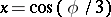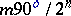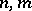The problem of dividing an angleinto three equal parts; one of the classical problems of Antiquity on ruler-and-compass construction. The solution of the problem of trisecting an angle reduces to finding rational roots of a cubic equation, where, which, in general, is not solvable by quadratic radicals. Thus, the problem of trisecting an angle cannot be solved by means of ruler and compass, as was proved in 1837 by P. Wantzel. However, such a construction is possible for angles, whereare integers, as well as by using other means and instruments of construction (for example, the Dinostratus quadratrix or the conchoid).